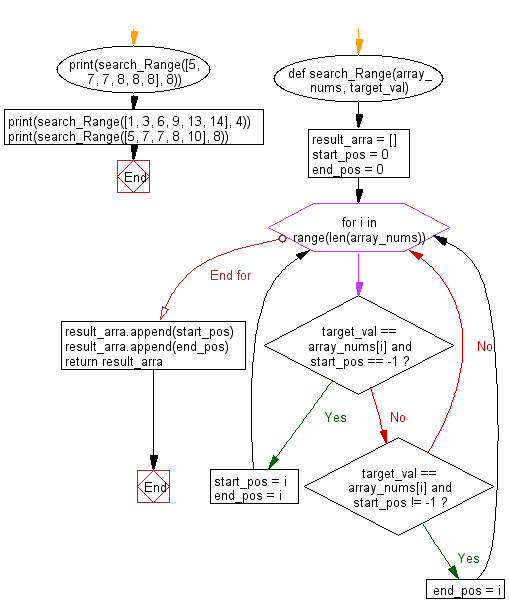﻿ Python: Find the starting and ending position of a given value in a given array of integers, sorted in ascending order - w3resource# Python: Find the starting and ending position of a given value in a given array of integers, sorted in ascending order

## Python Basic - 1: Exercise-76 with Solution

Write a Python program to find the starting and ending position of a given value in a given array of integers, sorted in ascending order.
If the target is not found in the array, return [0, 0].
Input: [5, 7, 7, 8, 8, 8] target value = 8
Output: [0, 5]
Input: [1, 3, 6, 9, 13, 14] target value = 4
Output: [0, 0]

Sample Solution:

Python Code:

``````def search_Range(array_nums, target_val):
result_arra = []
start_pos = 0
end_pos = 0
for i in range(len(array_nums)):
if target_val == array_nums[i] and start_pos == -1:
start_pos = i
end_pos = i
elif target_val == array_nums[i] and start_pos != -1:
end_pos = i
result_arra.append(start_pos)
result_arra.append(end_pos)
return result_arra
print(search_Range([5, 7, 7, 8, 8, 8], 8))
print(search_Range([1, 3, 6, 9, 13, 14], 4))
print(search_Range([5, 7, 7, 8, 10], 8))
``````

Sample Output:

```[0, 5]
[0, 0]
[0, 3]
```

Flowchart:Python Code Editor:

Have another way to solve this solution? Contribute your code (and comments) through Disqus.

What is the difficulty level of this exercise?

Test your Programming skills with w3resource's quiz.

﻿

## Python: Tips of the Day

How to make a flat list out of list of lists?

Given a list of lists l

```flat_list = [item for sublist in l for item in sublist]
```

which means:

```flat_list = []
for sublist in l:
for item in sublist:
flat_list.append(item)
```

is faster than the shortcuts posted so far. (l is the list to flatten.) Here is the corresponding function:

flatten = lambda l: [item for sublist in l for item in sublist]

As evidence, you can use the timeit module in the standard library:

```\$ python -mtimeit -s'l=[[1,2,3],[4,5,6], , [8,9]]*99' '[item for sublist in l for item in sublist]'
10000 loops, best of 3: 143 usec per loop
\$ python -mtimeit -s'l=[[1,2,3],[4,5,6], , [8,9]]*99' 'sum(l, [])'
1000 loops, best of 3: 969 usec per loop
\$ python -mtimeit -s'l=[[1,2,3],[4,5,6], , [8,9]]*99' 'reduce(lambda x,y: x+y,l)'
1000 loops, best of 3: 1.1 msec per loop
```

Explanation: the shortcuts based on + (including the implied use in sum) are, of necessity, O(L**2) when there are L sublists -- as the intermediate result list keeps getting longer, at each step a new intermediate result list object gets allocated, and all the items in the previous intermediate result must be copied over (as well as a few new ones added at the end). So, for simplicity and without actual loss of generality, say you have L sublists of I items each: the first I items are copied back and forth L-1 times, the second I items L-2 times, and so on; total number of copies is I times the sum of x for x from 1 to L excluded, i.e., I * (L**2)/2.

The list comprehension just generates one list, once, and copies each item over (from its original place of residence to the result list) also exactly once.

Ref: https://bit.ly/3dKsNTR